# Complex bandstructure of Si(100)¶

Version: 2015.1

In this tutorial you will calculate the complex bandstructure of a silicon crystal along the (100) direction.

In particular you will:

1. create the Si(100) surface;
2. set up and run the calculation;
3. plot the complex bandstructure (3D and 2D).## Background¶

In a periodic solid the eigenstates $$\psi_{n{\bf k}}$$ of the Schrödinger equation $$H \psi_{n{\bf k}} = E_{n{\bf k}} S \psi_{n{\bf k}}$$, ($$S$$ is the overlap matrix) can be written as $$\psi_{n{\bf k}}({\bf r}) = e^{-i {\bf k}\cdot {\bf r}} U_{n{\bf k}}({\bf r})$$, where $$U_{n{\bf k}}({\bf r})$$ is a periodic function with the same periodicity as the crystal itself. In a usual bandstructure calculation, the wave vector $${\bf k}$$ is a real number, and by solving the Schrödinger equation above for various fixed values of $${\bf k}$$ (typically located along different symmetry lines in the first Brillouin zone) an eigenproblem is defined, from which the eigenenergies $$E_{n{\bf k}}$$ (i.e. the bandstructure) can be determined.

When calculating the complex bandstructure another approach is taken [CS82]. Instead the energy $$E$$ is fixed, and the values of $${\bf k}$$ which solve the Schrödinger equation are sought. Such solutions will be found with both real and complex $${\bf k}$$; the solutions with a real $${\bf k}$$ are the usual Bloch states, while the solutions with an imaginary part are exponentially decaying in one direction and increasing in the other. Such solutions cannot exist in a bulk material, and so they are normally ignored in a bandstructure calculation. They may however exist at a surface or interface, and they give information about how electronic states decay in the material, for instance through a thin tunneling barrier.

Note

You will primarily use the graphical user interface QuantumATK for setting up and analyzing the results. If you are new to QuantumATK, it is recommended to go through the Basic QuantumATK Tutorial.

The calculations in this tutorial will be performed using the semi-empirical models of QuantumATK. A complete description of all the parameters, and in many cases a longer discussion about their physical relevance, can be found in the ATK Reference Manual. In particular, the Reference Manual entry for complex bandstructure calculations is of relevance: ComplexBandstructure.

## Si(100) surface¶

Start QuantumATK and create a new project named “ComplexBandstructure”. Then click Open and launch the Builder via the iconon the toolbar.

In the Builder, click Add ‣ From Database and locate the “Silicon (alpha)” structure. Double-click the line to add the structure to the Stash, or click the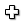icon in the lower right-hand corner.

Unfold Builders from the plugin panel and open the Surface (Cleave) tool, and follow the next steps to set up the Si(100) surface:

1. Keep the default Miller indices (100) and click Next.
2. Keep the default lattice vectors and click Next.
3. Select Periodic (bulk-like) for the out-of-plane cell vector.
4. Set the thickness of the surface to 1 layer.
5. Click Finish to add the Si(100) surface structure to the Stash.With these settings we use a surface representation with a minimum number of layers in order to reduce the calculation time and avoid zone folding.

Note

The cleave plane – in the present case (100) – is always spanned by the two first unit cell vectors, $${\bf}$$ and $${\bf B}$$. In the “electrode” mode, the normal to this plane coincides with $${\bf C}$$, the third unit cell vector, but in the “bulk-like” mode this is not the case. In QuantumATK the complex bandstructure is always computed along the third reciprocal vector, $${\bf g}_C$$, which is of course parallel to $${\bf A} \times {\bf B}$$. With the present geometry you will therefore obtain the complex bandstructure along (100).

## Complex bandstructure calculation¶

Send the structure to the Script Generator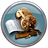using the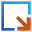icon in the lower right-hand corner of the Builder and follow the next steps:

1. Add aNew Calculator block.
2. Add theAnalysis ‣ ComplexBandStructure block.
3. Change the default output filename to si_100_cbs.hdf5.Next, open the insertedNew Calculator block (double-click it), and set up a tight-binding calculation:

1. Select the ATK-SE: Extended Hückel calculator.
2. Set the k-point sampling to 5x5x5.
3. Under Huckel Basis set, select the Cerda.Silicon [diamond GW] basis set. This basis set has been fitted to GW calculations, and gives an excellent description of the bandstructure, including the size of the band gap.
4. Close the dialogue by clicking OK.

Next, open theComplexBandstructure analysis block, and edit it:

• Set the energy range to -15 to 10 eV with 501 points.Note

The projection of $${\bf k}$$ on the cleave plane is kept constant in the complex andstructure calculation, and the value of the projection is specified by the $$(k_A,k_B)$$ parameters, which are given in units of the two first reciprocal lattice vectors, $${\bf g}_A$$ and $${\bf g}_B$$. Therefore, the obtained solutions will lie along the third reciprocal lattice vector, $${\bf g}_C$$, which is parallel to the cleave plane normal. A new set of solutions $$k_C+i\kappa_C$$ is obtained for each value of $$(k_A,k_B)$$.

Please note that $$k_C$$ is therefore the real part of the complex bandstructure, while $$kappa_C$$ is the complex part.

You have now finished the Python script. Save it as si_100_cbs.py and send it to the Job Manager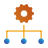for execution. It should only take a few minutes for this small system. If needed, you can also download the script here: si_100_cbs.py.

Tip

This type of calculation parallelizes extremely well, so for larger structures it is warmly recommended to execute the script in parallel.

## Analysing the results¶

The file si_100_cbs.hdf5 should now have appeared on the QuantumATK LabFloor. It contains the saved Hückel calculation and the ComplexBandstructure analysis object:ComplexBandstructure

Select that analysis object and click Show 2D Plot tool from the right-hand side plugins panel. A window with a plot of the complex bandstructure pops up. Zoom in a bit to filter out the solutions with large values of the complex part $$\kappa_C$$, since these are not really relevant:Fig. 46 Si(100) bandstructure. The right-hand part of the plot shows the real bands – note the indirect band gap of ~1.2 eV. The left-hand part of the plot shows the complex bands, plotted against the imaginary part, $$\kappa_C$$. The bands with a small imaginary part are the most important ones, since they are less damped if you should try to tunnel electrons through a thin slice of silicon in the (100) direction.

Note

In the plot above, the solutions $$\kappa_C$$ are given in reciprocal Cartesian coordinates, to make it easier to compare different structures. For the real part of the bandstructure, on the other hand, the solutions $$k_C$$ are normalized by $$L$$, the layer separation perpendicular to the cleave plane. In the case of a fcc crystal cleaved along (100), the layer separation is $$L=a/2$$, where $$a$$ is the lattice constant. The layer separation can be extracted from the ComplexBandstructure object using the layerSeparation() method, see ComplexBandstructure.

## 3D and 2D visualizations¶

In the complex part of the bandstructure, the solutions actually have a real part too. Thus, they could be visualized in a three-dimensional plot, $$(x,y,z)=(k_C, \kappa_C, E)$$. This is possible with the following script. Open the QuantumATK Editor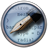and copy-paste the script and save it as 3D_plot.py. Make sure that the indentation is correct. Alternatively, you can simply download it here: 3D_plot.py.

  1 2 3 4 5 6 7 8 9 10 11 12 13 14 15 16 17 18 19 20 21 22 23 24 25 26 27 28 29 30 31 32 33 34 35 36 37 38 39 40 41 42 43 44 45 46 47 48 49 50 51 52 53 54 from QuantumATK import * from mpl_toolkits.mplot3d import Axes3D import matplotlib.pyplot as plt import math # Read the complex bandstructure object from the NC file cbs = nlread('si_100_cbs.nc', ComplexBandstructure)[-1] energies = cbs.energies().inUnitsOf(eV) k_real, k_complex = cbs.evaluate() L = cbs.layerSeparation() fig = plt.figure() ax = fig.add_subplot(111, projection='3d') # First plot the real bands kvr = numpy.array([]) e = numpy.array([]) # Loop over energies, and pick those where we have solutions for (j, energy) in enumerate(energies): k = k_real[j]*L/math.pi if len(k)>0: e = numpy.append(e,[energy,]*len(k)) kvr = numpy.append(kvr,k) # Plot the bands with red ax.scatter([0.0,]*len(kvr), kvr, e, c='r', marker='o', linewidths=0, s=10) # Next plot the complex bands kvr = [] kvi = [] e = [] # Again loop over energies and pick solutions for (j, energy) in enumerate(energies): if len(k_complex[j])>0: for x in numpy.array(k_complex[j]*L/math.pi): kr = numpy.abs(x.real) ki = -numpy.abs(x.imag) # Discard rapidly decaying modes which clutter the plot if ki>-0.3: e += [energy] kvr += [kr] kvi += [ki] # Plot the complex bands with blue ax.scatter(kvi, kvr, e, c='b', marker='o', linewidths=0, s=10) # Put on labels ax.set_xlabel('$\kappa$ (1/Ang)') ax.set_ylabel('$kL/\pi$') ax.set_zlabel('Energy / eV') plt.show() 

Execute the script using the Job Manageror from a Terminal. The plot will resemble the figure below, but your points will be more scattered, since the figure below was produced from a Hückel calculation with 10,001 energy points instead of 501.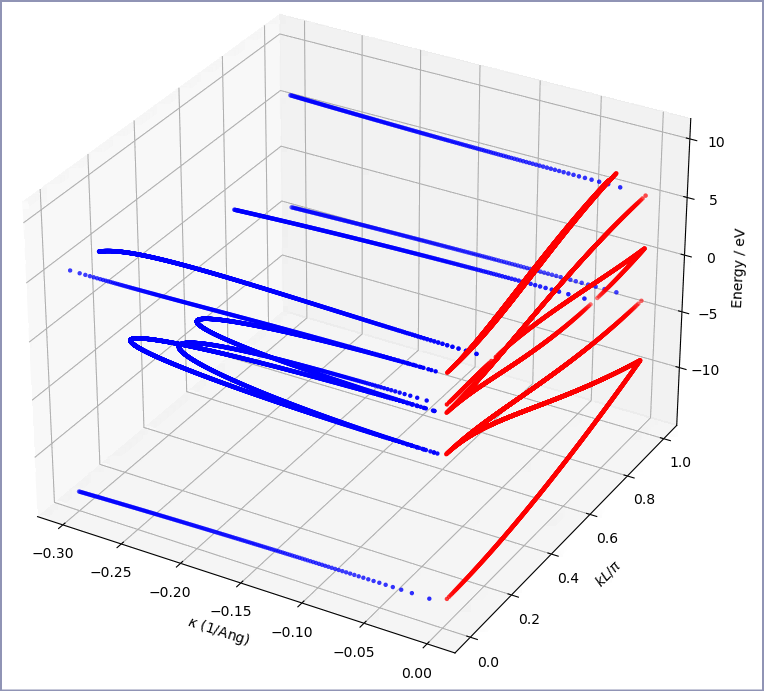Fig. 47 3D visualization of the complex bandstructure of Si(100). The real bands are plotted with red dots and the complex part in blue. Note how the complex bands connect with the real bands.

Another way to visualize the real part of the complex bandstructure is using colors. The script 2D_plot.py does this (you can see the script below). It is a bit complicated towards the end, but that part is just for placing the colorbar, and could be omitted.Fig. 48 2D visualization of the complex bandstructure. Note how the color-coding of the k-values applies both to the real and the complex parts of the bandstructure, which makes it easier to see where the complex bands are attached to the real ones.

The “forest” of complex bands with a rather large value of $$\kappa$$ are not usually seen in tight-binding simulations. However, for DFT and Hückel, the basis sets are larger, so there are more complex bands as well, connecting unoccupied levels with various valence bands.

  1 2 3 4 5 6 7 8 9 10 11 12 13 14 15 16 17 18 19 20 21 22 23 24 25 26 27 28 29 30 31 32 33 34 35 36 37 38 39 40 41 42 43 44 45 46 47 48 49 50 51 52 53 54 55 56 57 58 59 60 61 62 63 64 65 66 67 68 69 70 from QuantumATK import * import matplotlib.pyplot as plt import math # Read the complex bandstructure object from the NC file cbs = nlread('si_100_cbs.nc', ComplexBandstructure)[-1] energies = cbs.energies().inUnitsOf(eV) k_real, k_complex = cbs.evaluate() ax = plt.axes() cmap="Spectral" # First plot the real bands kvr = numpy.array([]) e = numpy.array([]) for (j, energy) in enumerate(energies): k = k_real[j]*cbs.layerSeparation()/math.pi if len(k)>0: e = numpy.append(e,[energy,]*len(k)) kvr = numpy.append(kvr,k) # Plot ax.scatter(kvr, e, c=numpy.abs(kvr), cmap=cmap, marker='o', linewidths=0, s=10) # Next plot the complex bands kvr = numpy.array([]) kvi = numpy.array([]) e = numpy.array([]) for (j, energy) in enumerate(energies): if len(k_complex[j])>0: kr = [numpy.abs(x.real) for x in k_complex[j]] ki = [numpy.abs(x.imag) for x in k_complex[j]] e = numpy.append(e,[energy,]*len(kr)) kvr = numpy.append(kvr,kr) kvi = numpy.append(kvi,ki) # Plot with color depending on the imaginary part (corresponding to real k-points) sc = ax.scatter(-kvi, e, c=kvr, cmap=cmap, marker='o', linewidths=0, s=10) # Put on labels and decorations ax.axvline(0,color='b') ax.grid(True, which='major') ax.set_xlim(-1, 1) ax.set_ylim(-15, 10) ax.annotate('$\kappa$ (1/Ang)', xy=(0.25,-0.07), xycoords="axes fraction", ha="center") ax.annotate('$kL / \pi$', xy=(0.75,-0.07), xycoords="axes fraction", ha="center") ax.set_ylabel('Energy / eV') # Add a colorbar fig = plt.gcf() x1, x2, y1, y2 = 0., 1, ax.get_ylim(), ax.get_ylim()+1 trans = ax.transData + fig.transFigure.inverted() ax_x1, ax_y1 = trans.transform_point([x1, y1]) ax_x2, ax_y2 = trans.transform_point([x2, y2]) ax_dx, ax_dy = ax_x2 - ax_x1, ax_y2 - ax_y1 cmap_axes = plt.axes([ax_x1, ax_y1, ax_dx, ax_dy]) a = numpy.outer(numpy.arange(0,1,0.01),numpy.ones(10)).transpose() cmap_plt = plt.imshow(a,aspect='auto',cmap=plt.get_cmap(cmap),origin=(0,0)) ax = plt.gca() ax.set_xticks([]) ax.set_yticks([]) ax.set_xticklabels([]) ax.set_yticklabels([]) plt.show() 

Hint

You may note that there are “gaps” in the visualizations. The reason is that, unlike a normal bandstructure plot, the data is not plotted with lines, but with dots. In a standard bandstructure plot you can – with some level of confidence – define “bands”, which continuously run between the symmetry points (only band crossings can create some small problems). In the present case, the number of solutions is first of all different at each energy (particularly on the complex side), and depending on the density of the energy sampling, you may not hit a particular band close to points where the bands are very flat (heavy effective mass). This can to some extent be alleviated by increasing the number of energy points.